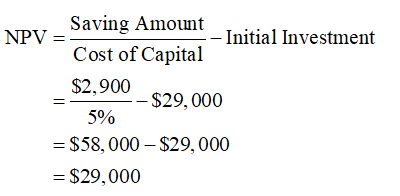# f you insulate your office for \$29,000, you will save \$2,900 a year in heating expenses. These savings will last forever. a. What is the NPV of the investment when the cost of capital is 5%? 10%?b. What is the IRR of the investment? (Enter your answer as a whole percent.)c. What is the payback period on this investment?

Question
12 views

f you insulate your office for \$29,000, you will save \$2,900 a year in heating expenses. These savings will last forever.

a. What is the NPV of the investment when the cost of capital is 5%? 10%?

b. What is the IRR of the investment? (Enter your answer as a whole percent.)

c. What is the payback period on this investment?

check_circle

Step 1

a.

Given,

Saving Amount per Year = \$2,900

Investment Amount = \$29,000

NPV at Cost of Capital of 5%:

The calculation of the NPV of the investment at the cost of capital at 5% is as follows:Hence, NPV is \$29,000 when the cost of capital is 5%.

NPV at Cost of Capital of 10%:

The calculation of the NPV of the investment at the cost of capital at 10% is as follows:Hence, NPV is \$0 when the cost of capital is 10%.

Step 2

The rate at which the NPV is zero is termed as IRR and in this investment, the NPV is ze...

### Want to see the full answer?

See Solution

#### Want to see this answer and more?

Solutions are written by subject experts who are available 24/7. Questions are typically answered within 1 hour.*

See Solution
*Response times may vary by subject and question.
Tagged in